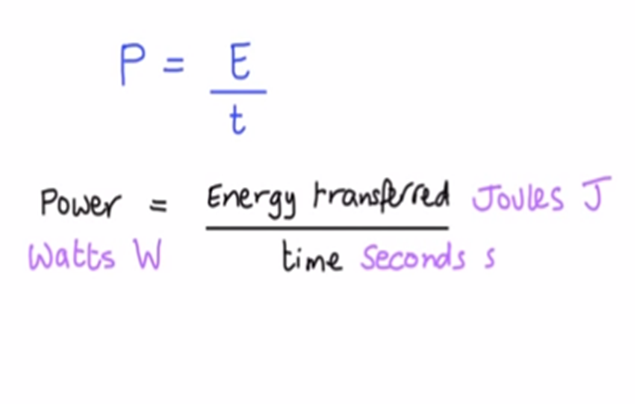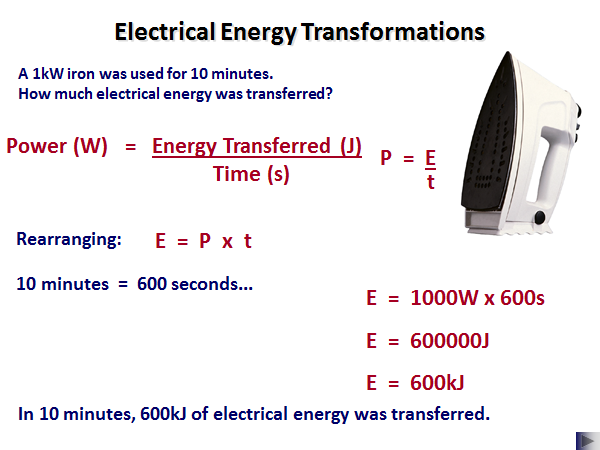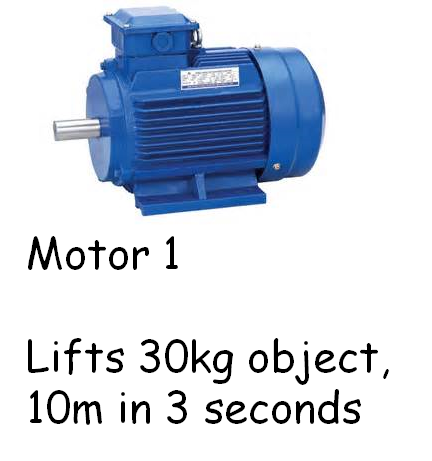# Power

## What Is Power

Power is defined as _the rate at which energy is transferred. _

_﻿﻿_This means that the higher the power, the quicker energy is transferred.

## Power Equation

The equation for power is:This is sometimes written as: P = W/t

In this case W = work done and is also measured in Joules (J).

## Using the Power EquationNotice how the minutes was changed into seconds. For all the physics equations, we must make sure that we use seconds and not minutes or hours.

Also, the 1kW was also converted into 1000W. To convert from kW to W you multiply by 1000.

## 5Mark Questions

Calculate the power of Motor 1.This is an example of a 5 mark question as two equations have to be used.

It is clear from the question that P = E/t must be used.

We are missing E, energy.

The question include the mass of the object and the height it is lifted. The only equation that fits these variables is Ep = m g h

Therefore: Ep = 30 x 10 x 10 = 3000J

We can substitute this value into the power equation:

P = 3000/3 = 1000W or 1kW

What is the energy supplied to an appliance that is used for 18 hours and has a power usage of 0.1kW? (in Joules)